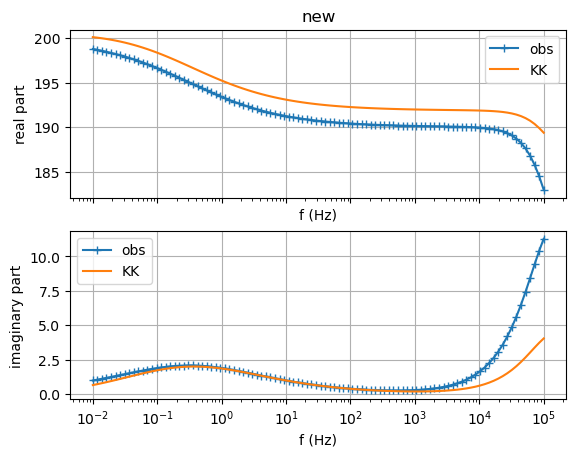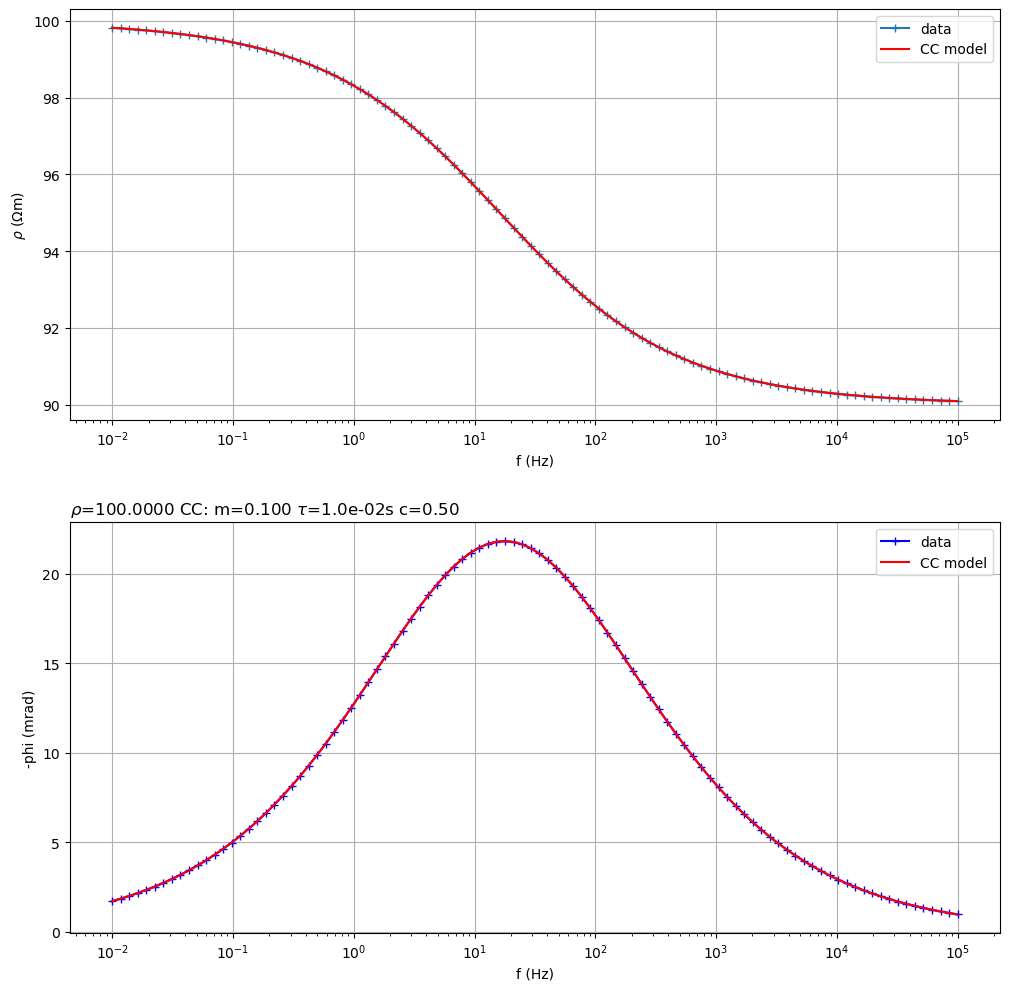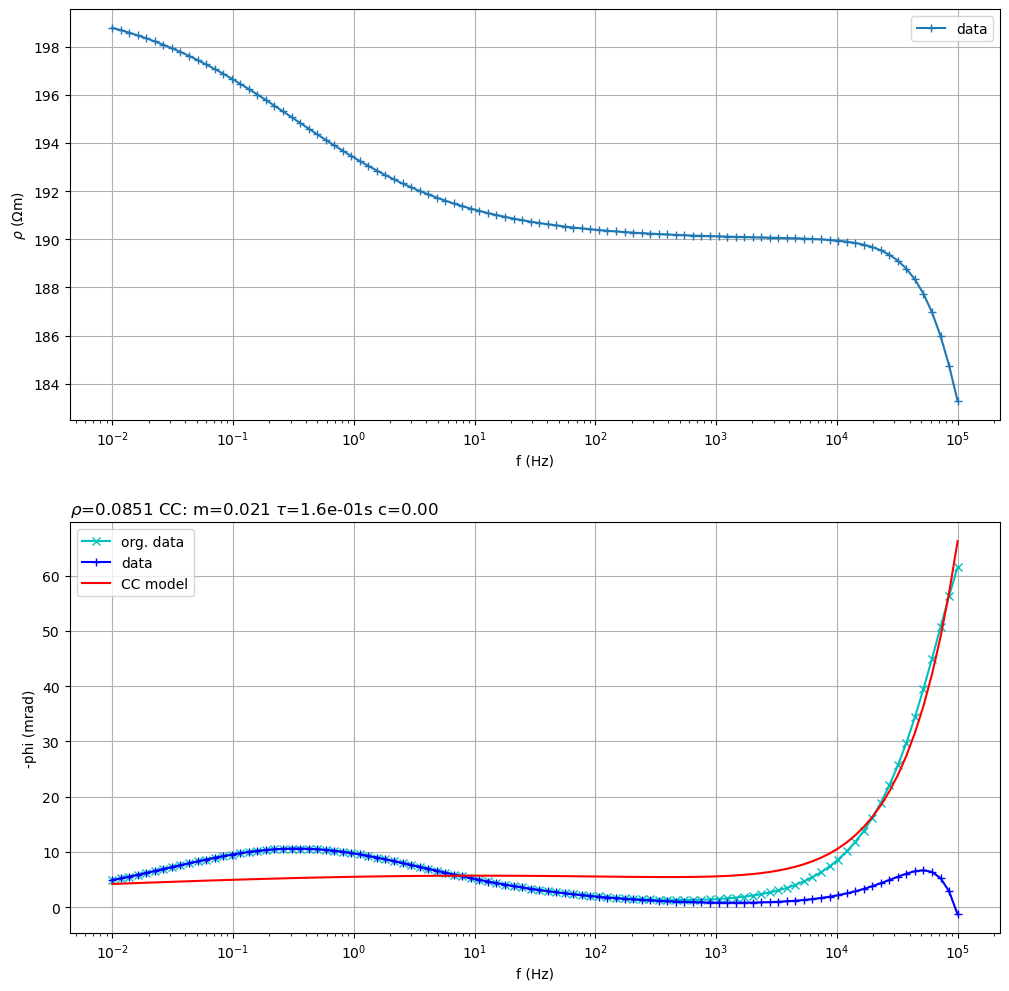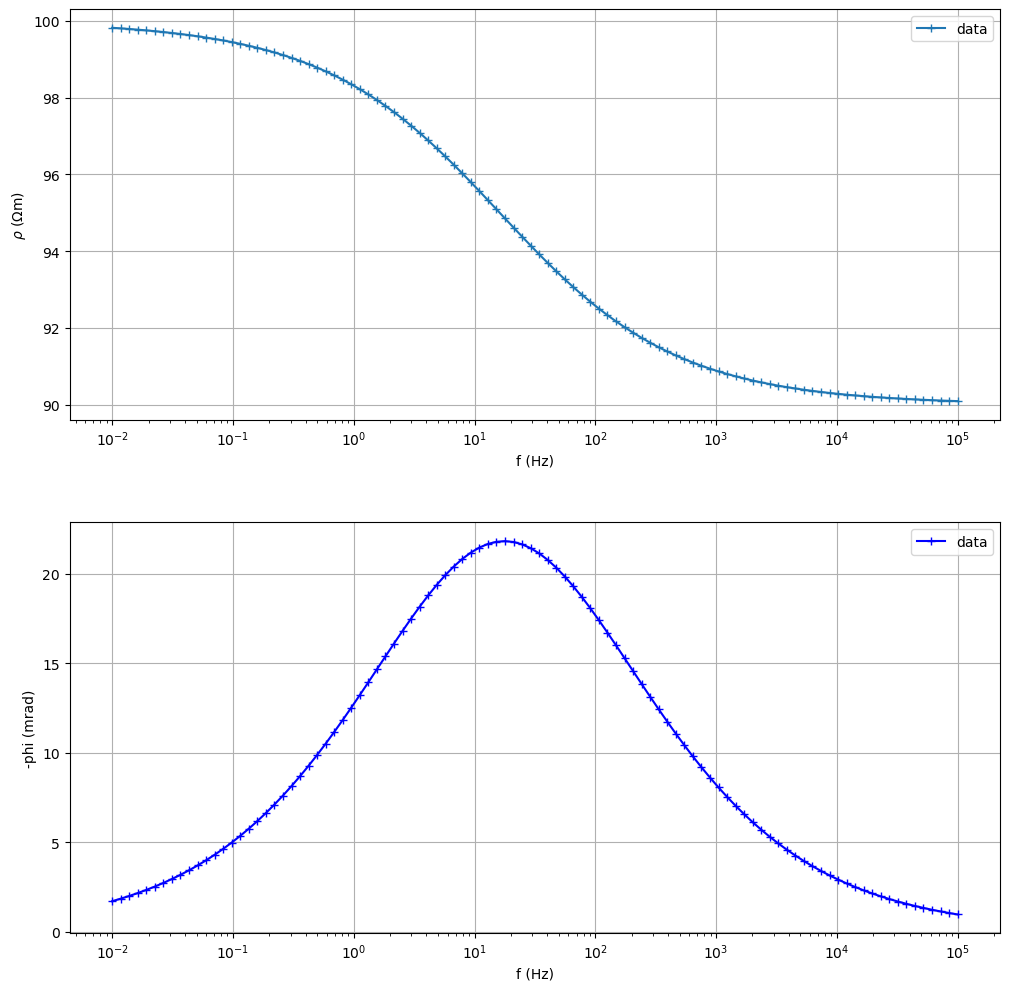# Fitting SIP signatures#

This example highlights some of the capabilities of pyGimli to analyze spectral induced polarization (SIP) signatures.

Author: Maximilian Weigand, University of Bonn

Import pyGIMLi and related stuff for SIP Spectra

from pygimli.physics.SIP import SIPSpectrum, modelColeColeRho
import numpy as np
import pygimli as pg


1. Generate synthetic data with a Double-Cole-Cole Model and initialize a SIPSpectrum object

f = np.logspace(-2, 5, 100)
Z1 = modelColeColeRho(f, rho=1, m=0.1, tau=0.5, c=0.5)
Z2 = modelColeColeRho(f, rho=1, m=0.25, tau=1e-6, c=1.0)

rho0 = 100 # (Ohm m)
Z = rho0 * (Z1 + Z2)

sip = SIPSpectrum(f=f, amp=np.abs(Z), phi=-np.angle(Z))
# Note the minus sign for the phases: we need to provide -phase[rad]

sip.showData()
sip.showDataKK()  # check Kramers-Kronig relations

••(<Figure size 640x480 with 2 Axes>, array([<matplotlib.axes._subplots.AxesSubplot object at 0x7fa074ae5340>,
<matplotlib.axes._subplots.AxesSubplot object at 0x7fa06fdd1d90>],
dtype=object))

1. Fit a Cole-Cole model from synthetic data

Z = modelColeColeRho(f, rho=100, m=0.1, tau=0.01, c=0.5)
# TODO data need some noise

sip = SIPSpectrum(f=f, amp=np.abs(Z), phi=-np.angle(Z))
sip.fitColeCole(useCond=False, verbose=False)  # works for both rho and sigma models
sip.showAll()(<Figure size 1200x1200 with 2 Axes>, array([<matplotlib.axes._subplots.AxesSubplot object at 0x7fa06fa44a00>,
<matplotlib.axes._subplots.AxesSubplot object at 0x7fa074d5bd00>],
dtype=object))

1. Fit a double Cole-Cole model

f = np.logspace(-2, 5, 100)
Z1 = modelColeColeRho(f, rho=1, m=0.1, tau=0.5, c=0.5)
Z2 = modelColeColeRho(f, rho=1, m=0.25, tau=1e-6, c=1.0)

rho0 = 100 #(Ohm m)
Z = rho0 * (Z1 + Z2)

# TODO data need some noise
sip = SIPSpectrum(f=f, amp=np.abs(Z), phi=-np.angle(Z))
sip.fitCCEM(verbose=False) # fit an SIP Cole-Cole term and an EM term (also Cole-Cole)
sip.showAll()(<Figure size 1200x1200 with 2 Axes>, array([<matplotlib.axes._subplots.AxesSubplot object at 0x7fa0747ffeb0>,
<matplotlib.axes._subplots.AxesSubplot object at 0x7fa05545c880>],
dtype=object))

1. Fit a Cole-Cole model to

f = np.logspace(-2, 5, 100)
Z = modelColeColeRho(f, rho=100, m=0.1, tau=0.01, c=0.5)
sip = SIPSpectrum(f=f, amp=np.abs(Z), phi=-np.angle(Z))

sip.showAll()
sip.fitDebyeModel(new=True, showFit=True)
pg.wait()

••ARMS= 0.0003210339516863225 RRMS= 0.32922929218525215


Note

This tutorial was kindly contributed by Maximilian Weigand (University of Bonn). If you also want to contribute an interesting example, check out our contribution guidelines https://www.pygimli.org/contrib.html.

Gallery generated by Sphinx-Gallery